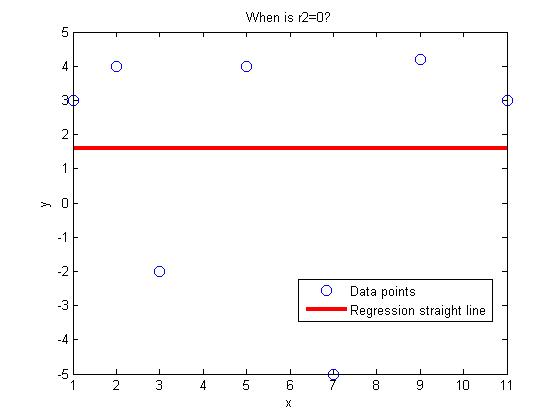# In regression, when is coefficient of determination zero

The coefficient of determination is a measure of how much of the original uncertainty in the data is explained by the regression model.

The coefficient of determination, $latex r^2$ is defined as

$latex r^2$=$latex \frac{S_t-S_r}{S_r}$

where

$latex S_t$ = sum of the square of the differences between the y values and the average value of y

$latex S_r$ = sum of the square of the residuals, the residual being the difference between the observed and predicted values from the regression curve.

The coefficient of determination varies between 0 and 1. The value of the coefficient of determination of zero means that no benefit is gained by doing regression. When can that be?

One case comes to mind right away – what if you have only one data point. For example, if I have only one student in my class and the class average is 80, I know just from the average of the class that the student’s score is 80. By regressing student score to the number of hours studied or to his GPA or to his gender would not be of any benefit. In this case, the value of the coefficient of determination is zero.

What if we have more than one data point? Is it possible to get the coefficient of determination to be zero?

The answer is yes. Look at the following data pairs (1,3), (3,-2), (5,4), (7,-5), (9,4.2), (11,3), (2,4). If one regresses this data to a general straight line

y=a+bx,

one gets the regression line to be

y=1.6In fact, 1.6 is the average value of the given y values. Is this a coincidence? Because the regression line is the average of the y values, $latex S_t=S_r$, implying $latex r^2=0$

QUESTIONS

1. Given (1,3), (3,-2), (5,4), (7,a), (9,4.2), find the value of a that gives the coefficient of determination, $latex r^2=0$. Hint: Write the expression for $latex S_r$ for the regression line $latex y=mx+c$. We now have three unknowns, m, c and a. The three equations then are $latex \frac{\partial S_r} {\partial m} =0$, $latex \frac{\partial S_r} {\partial c} =0$ and $latex S_t=S_r$.
2. Show that if n data pairs $latex (x_1,y_1)……(x_n,y_n)$ are regressed to a straight line, and the regression straight line turns out to be a constant line, then the equation of the constant line is always y=average value of the y-values.

This post is brought to you by Holistic Numerical Methods: Numerical Methods for the STEM undergraduate at http://nm.mathforcollege.com

1.Russ Aveney says:
1.Autar Kaw says: